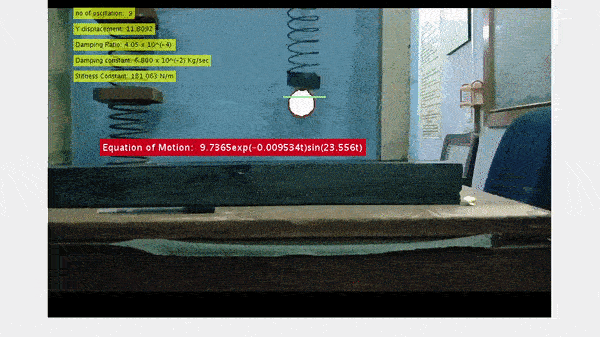# Harmonic Motion AnalyzerSample Result

•Aimed at estimation equation of motion of a target-object video is selected to analyze its motion.
•Aimed at retrieving data associated with the motion of target-object using elementary mathematics.
This project was made for TechnoSeason, 2017 and was awarded first prize.

### Abstract

Simple harmonic motion can serve as a mathematical model for a variety of motions, such as the oscillation of a spring. Intending to learn computer vision and MATLAB, I worked on analyzing the motion of a target-object undergoing a damped harmonic motion. The target-object was separated from the background using color thresholding and estimated as a point object. Coordinates of this point were recorded and used to estimate the parameters associated with the mathematical model of the system like maximum displacement, mean position, the velocity at different time instants. A mathematical model was estimated by fitting a curve to the recorded data using MATLAB Curve Fitting Toolbox.##### Khush Agrawal
###### Senior Year Undergraduate Student

Reinforcement Learning